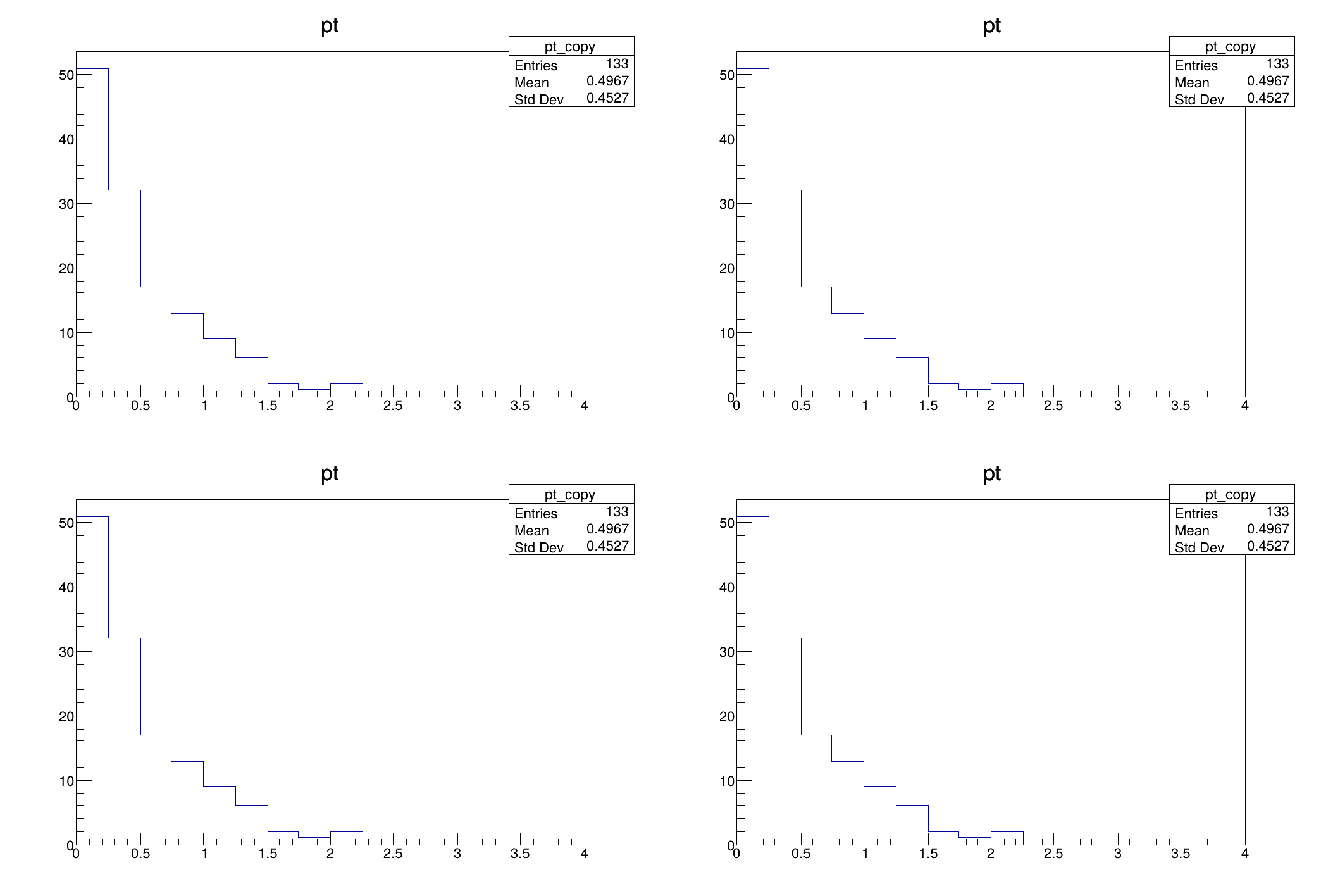ROOT   Reference Guide
Searching...
No Matches
df017_vecOpsHEP.C File Reference

## Detailed DescriptionUse RVecs to plot the transverse momentum of selected particles.

This tutorial shows how VecOps can be used to slim down the programming model typically adopted in HEP for analysis. In this case we have a dataset containing the kinematic properties of particles stored in individual arrays. We want to plot the transverse momentum of these particles if the energy is greater than 100 MeV.

auto filename = gROOT->GetTutorialDir() + "/dataframe/df017_vecOpsHEP.root";
auto treename = "myDataset";
using namespace ROOT;
{
TH1F h("pt", "pt", 16, 0, 4);
while (tr.Next()) {
for (auto i=0U;i < px.GetSize(); ++i) {
if (E[i] > 100) h.Fill(sqrt(px[i]*px[i] + py[i]*py[i]));
}
}
h.DrawCopy();
}
void WithRDataFrame()
{
RDataFrame f(treename, filename.Data());
auto CalcPt = [](RVecD &px, RVecD &py, RVecD &E) {
for (auto i=0U;i < px.size(); ++i) {
if (E[i] > 100) {
v.emplace_back(sqrt(px[i]*px[i] + py[i]*py[i]));
}
}
return v;
};
f.Define("pt", CalcPt, {"px", "py", "E"})
.Histo1D<RVecD>({"pt", "pt", 16, 0, 4}, "pt")->DrawCopy();
}
void WithRDataFrameVecOps()
{
RDataFrame f(treename, filename.Data());
auto CalcPt = [](RVecD &px, RVecD &py, RVecD &E) {
auto pt = sqrt(px*px + py*py);
return pt[E>100];
};
f.Define("good_pt", CalcPt, {"px", "py", "E"})
.Histo1D<RVecD>({"pt", "pt", 16, 0, 4}, "good_pt")->DrawCopy();
}
void WithRDataFrameVecOpsJit()
{
RDataFrame f(treename, filename.Data());
f.Define("good_pt", "sqrt(px*px + py*py)[E>100]")
.Histo1D({"pt", "pt", 16, 0, 4}, "good_pt")->DrawCopy();
}
{
// We plot four times the same quantity, the key is to look into the implementation
// of the functions above.
auto c = new TCanvas();
c->Divide(2,2);
c->cd(1);
c->cd(2);
WithRDataFrame();
c->cd(3);
WithRDataFrameVecOps();
c->cd(4);
WithRDataFrameVecOpsJit();
}
#define f(i)
Definition RSha256.hxx:104
#define c(i)
Definition RSha256.hxx:101
#define h(i)
Definition RSha256.hxx:106
Option_t Option_t TPoint TPoint const char GetTextMagnitude GetFillStyle GetLineColor GetLineWidth GetMarkerStyle GetTextAlign GetTextColor GetTextSize void char Point_t Rectangle_t WindowAttributes_t Float_t Float_t Float_t Int_t Int_t UInt_t UInt_t Rectangle_t Int_t Int_t Window_t TString Int_t GCValues_t GetPrimarySelectionOwner GetDisplay GetScreen GetColormap GetNativeEvent const char const char dpyName wid window const char font_name cursor keysym reg const char only_if_exist regb h Point_t winding char text const char depth char const char Int_t count const char ColorStruct_t color const char filename
#define gROOT
Definition TROOT.h:406
reference emplace_back(ArgTypes &&...Args)
Definition RVec.hxx:920
ROOT's RDataFrame offers a modern, high-level interface for analysis of data stored in TTree ,...
The Canvas class.
Definition TCanvas.h:23
A ROOT file is composed of a header, followed by consecutive data records (TKey instances) with a wel...
Definition TFile.h:54
1-D histogram with a float per channel (see TH1 documentation)}
Definition TH1.h:577
An interface for reading collections stored in ROOT columnar datasets.
A simple, robust and fast interface to read values from ROOT columnar datasets such as TTree,...